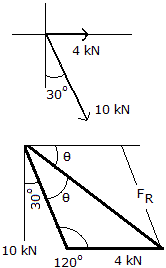Engineering Mechanics - Force Vectors - Discussion

Discussion :: Force Vectors - General Questions (Q.No.23)

23.Determine the magnitudes of the resultant force and its direction measured from the positive x axis.

 [A]. R = 12.49 kN,= 43.9° CW [B]. R = 13.6 kN,= 21.5° CW [C]. R = 14.00 kN,= 60.0° CW [D]. R = 10.80 kN,= 68.2° CW

Explanation:

No answer description available for this question.

 Kailas said: (Jan 21, 2013) Fx=+4+10sin(30)=9. Fy=-10cos(30)=-8.66. R= in square root sum of Fx square and Fy square=12.48.

 Labeeq said: (Mar 7, 2017) What about the angle?